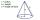# Volume of cone

Find the volume of a right circular cone-shaped building with a height of 9 cm and a radius base of 7 cm.

Result

V =  461.814 cm3

#### Solution:Leave us a comment of example and its solution (i.e. if it is still somewhat unclear...):

Showing 0 comments:Be the first to comment!#### To solve this example are needed these knowledge from mathematics:

Tip: Our volume units converter will help you with converion of volume units.

## Next similar examples:

1. Truncated coneCalculate the volume of a truncated cone with base radiuses r1=13 cm, r2 = 10 cm and height v = 8 cm.
2. Rotating coneCalculate volume of a rotating cone with base radius r=12 cm and height h=7 cm.
3. Rectangular prismIf i have a rectangular prism with a length of 1,000 cm, width of 30 cm and a height of 50 cm, what is the volume?
4. Volume of cubeSolve the volume of a cube with width 26cm .
5. Fire tankHow deep is the fire tank with the dimensions of the bottom 7m and 12m, when filled with 420 m3 of water?
6. Cylindrical tank 2If a cylindrical tank with volume is used 12320cm raised to the power of 3 and base 28cm is used to store water. How many liters of water can it hold?
7. Cube cornersThe wooden cube with edge 64 cm was cut in 3 corners of cube with edge 4 cm. How many cubes of edge 4 cm can be even cut?
8. The diagramThe diagram is a cone of radius 8cm and height 10cm. The diameter of the base is. ..
9. 22/7 circleCalculate approximately area of a circle with radius 20 cm. When calculating π use 22/7.
10. Circle - simpleThe circumference of a circle is 930 mm. How long in mm is its diameter?
11. Two circlesTwo circles with a radius 4 cm and 3 cm have a center distance 0.5cm. How many common points have these circles?
12. AnnulusThe radius of the larger circle is 8cm, the radius of smaller is 5cm. Calculate the contents of the annulus.
13. Bicycle wheelAfter driving 157 m bicycle wheel rotates 100 times. What is the radius of the wheel in cm?
14. Clock handsThe second hand has a length of 1.5 cm. How long does the endpoint of this hand travel in one day?
15. Cupcakes 2Susi has 25 cupcakes. She gives 4/5. How much does she have left?
16. DisjointHow many elements have union and intersection of two disjoint sets when the first have 1 and secodn 8 elements.
17. CircleWhat is the radius of the circle whose perimeter is 6 cm?